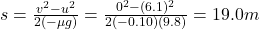## A steel ball of mass m1 = 1.1 kg and a cord of length of L = 1.9 m of negligible mass make up a simple pendulum that can pivot without frict

Question

A steel ball of mass m1 = 1.1 kg and a cord of length of L = 1.9 m of negligible mass make up a simple pendulum that can pivot without friction about the point O, as in the figure below. This pendulum is released from rest in a horizontal position, and when the ball is at its lowest point it strikes a block of mass m2 = 1.1 kg sitting at rest on a shelf. Assume that the collision is perfectly elastic and that the coefficient of kinetic friction between the block and shelf is 0.10.(a) What is the velocity of the block just after impact?(b) How far does the block slide before coming to rest (assuming that the shelf is long enough)?

in progress 0
5 months 2021-08-14T00:41:36+00:00 1 Answers 7 views 0

1. a) 6.1 m/s

b) 19.0 m

Explanation:

a)

Before the collision, the initial gravitational potential energy of the pendulum is converted into kinetic energy. So, we can write: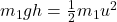where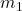is the mass of the steel ball attached to the pendulum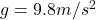is the acceleration due to gravity

h = 1.9 m is the initial height of the steel ball (since the pendulum is in horizontal position)

u is the velocity of the steel ball just before hitting the block

Solving for u,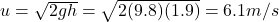After that, the steel ball collides elastically with the block, of mass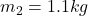The collision is elastic, this means that both total momentum and total kinetic energy are conserved, so we can write: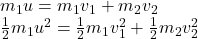where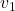is the final velocity of the steel ball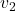is the final velocity of the block

Solving simultaneously the two equations, we  can find the final velocity of the block: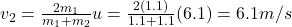Because the two objects have same mass, so the steel ball has transferred all its energy and momentum to the block.

b)

After the collision, the block slides with a certain deceleration given by the presence of the force of friction.

The force of friction on the block is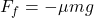where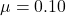is the coefficient of friction

m = 1.1 kg is the mass of the blockis the acceleration due to gravity

The acceleration of the block is therefore (Newton’s second law):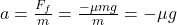Since the motion is a uniformly accelerated motion, we can use the suvat equation to determine the distance the block covers before coming to rest: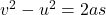where

v = 0 is the final velocity

u = 6.1 m/s is the initial velocity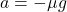is the acceleration

s is the stopping distance

Solving for s,